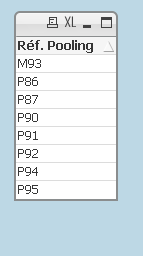# QlikView App Dev

Discussion Board for collaboration related to QlikView App Development.

Announcements
Our next Qlik Insider session will cover new key capabilities. Join us August 11th REGISTER TODAY
cancel
Showing results for
Did you mean:Contributor II

## pivot table expression

Hi,

Basically I am working on a pivot table. Can anyone help me to solve the following equation? it would very much be appreciated.

 Number of reference « pooling Pxxx» : Number of [Ref. Pooling] starting with P***
 Number of reference « Mono Mxxx» : Number of [Ref. Pooling] starting with M*** or
Number of [Ref. Pooling] with [Code raison] « camion « mono-industriel »
 Pooling rate (camions théoriques) = Number of [Ref. Pooling] starting with P*** / total
Number of reference (« pooling Pxxx + Mono Mxxx »)Labels (2)

• ### Pivot Tables

1 Solution

Accepted SolutionsContributor III

Hi,

For number 1 and 2 can done by this dimension. and tick 'Suppress When Value is Null' in the same tab.

=IF(LEFT([Ref. Pooling],1)='M','MXXX',
IF(LEFT([Ref. Pooling],1)='P','PXXX'))

For number 3, go to Presentation tab, Tick 'Show Partial Sums'

For the expression, put COUNT(DISTINCT [Ref. Pooling])

Thanks.

3 RepliesContributor III

Hi, I'm not very understand about your requirement but I hope below helps.

=IF(LEFT([No Of Reference],1)='M','MXXX',
IF(LEFT([No Of Reference],1)='P','PXXX',
[No Of Reference]))

For Expression, put COUNT(DISTINCT [No Of Reference])

Thanks.

JeffContributor II
Author

Hi jefwork,

the following are the ones I need to be solved.

1.Number of [Ref. Pooling] starting with P***

2.Number of [Ref. Pooling] starting with M***

3.Number of [Ref. Pooling] starting with P*** / total
Number of reference (Number of [Ref. Pooling] starting with P*** + Number of [Ref. Pooling] starting with M***)Contributor III

Hi,

For number 1 and 2 can done by this dimension. and tick 'Suppress When Value is Null' in the same tab.

=IF(LEFT([Ref. Pooling],1)='M','MXXX',
IF(LEFT([Ref. Pooling],1)='P','PXXX'))

For number 3, go to Presentation tab, Tick 'Show Partial Sums'

For the expression, put COUNT(DISTINCT [Ref. Pooling])

Thanks.Community Browser